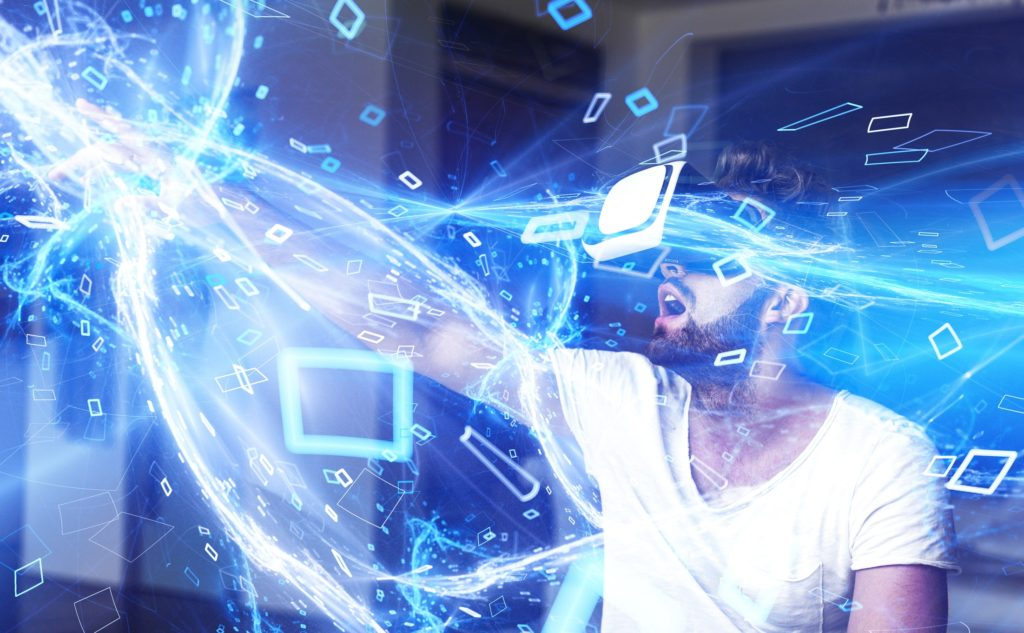# What is energy, and how does energy flow?

In the lessons, I regularly talk about energy, particularly the flow of energy through your body.

I know that energy is a complicated concept. It seems like everyone has a different idea on this subject.

As a physicist, I know that energy is nothing more than a number. Just like when you measure the temperature of your body (in my case 36.6 degrees Celsius), the number of degrees 36.6 is just a number. Physicists say the number 36.6 is the temperature of your “body heat”.

Or the number 120 is the value of the property ‘speed’ of my moving car on the highway (expressed in Km/hour😊).

Other examples:

So, energy is just a property. But of what?

Wikipedia states: “Energy is referred to as the ability or capacity to do work, or more broadly, the ability to effect change.”

Work is a measure of the effort done, or the effort expended by a power source when moving a mass. Is the power source a constant force F and the mass is displaced over a distance x then

Work = F . X

In short, you can say that labour (physical) is the amount of something that has taken place (or will take place).

On the other hand, energy is then an amount of something that could potentially be possible.

Since you can combine the different types of energy (electrical, thermal, gravitational, chemical, nuclear,..) into two basic types (potential and kinetic), you get the following scheme:

I understand that the previous has been described quite generally, without too much detail and depth. But even with this helicopter view, energy doesn’t seem like such a simple concept.

It gets even worse when we discuss the amount of energy and try to understand the energy flow.

Let me give an example of the electric current and energy flow with a simple light bulb (or better, an LED lamp 😊).

Based on the laws of electrodynamics, energy cannot flow in the same direction as the electric current. According to the Poynting vector, electric current will flow anywhere with both an electric field and a magnetic field. The electromagnetic field will flow in the direction of the Poynting Vector. The consequences will undoubtedly surprise you.

Poynting vector, a quantity describing the magnitude and direction of the flow of energy in electromagnetic waves. It is named after English physicist John Henry Poynting, who introduced it in 1884.

The Poynting vector S is defined as to be equal to the cross product (1/μ) E × B, where μ is the permeability of the medium through which the radiation passes, E is the amplitude of the electric field, and B is the amplitude of the magnetic field.

Instead of doing magic with mathematical formulas, I want to graphically represent the energy flow and electric current in simple animations.  These are based on Nick Lucid’s material and his fantastic blog on YouTube: “The Science Asylum”.

Take a simple example, where a battery lights up a light bulb.

#### The electric current.

If you connect both poles of a full battery, the electric current will flow from the positive pole to the negative.

#### Energy value.

Place a lamp between both poles of the battery, and the lamp will light up. By glowing the lamp, the energy in the battery will decrease.

#### Energy flow or Energy direction.

We now know the flow of electricity, and we know the electric fields are perpendicular to the magnetic fields (see Poynting’s formula). This will cause the battery to lose its energy in the direction PERPENDICULAR to the electric current direction!?! In this case, the energy will flow across the battery. The opposite happens with the lamp. This gives the following animation:

The same thing happens with alternating electric current.

What always surprises me is that this law also applies when the distance between battery and lamp are many kilometres apart (distance between A and B).

Even then, the battery gives away its energy to its immediate surroundings and many kilometres further, and the lamp absorbs the same energy. I still find this so amazing.

How does one energy know how much to give up and take upon the other?

The total energy always remains the same!!

Energy is such a fantastic thing.

Within our class, we will use Tai Chi to relax and even stretch our muscles. It is only in these muscle states that our body will absorb energy. In all other cases (e.g. muscle contraction), we will lose power. It is up to us to decide in which muscle state we want to live.

Scroll to Top